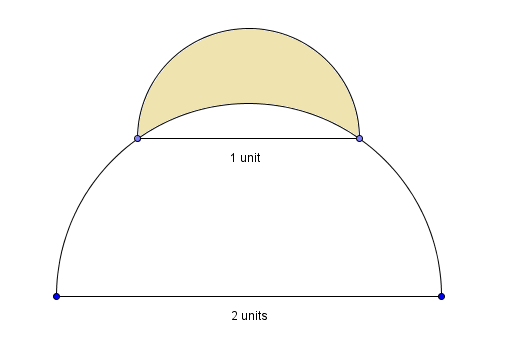# That looks like a moon!

Geometry Level 3A semi circle of diameter 1 unit sits at the top of a semi circle of diameter 2 units. the shaded region inside the the semi circle but outside the larger semi circle is called a lune. If the area of the lune is $\alpha$, then find the value of $\lfloor 1000\alpha\rfloor$

Note

• Assume that the diameters are parallel to each other.
×